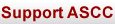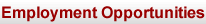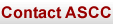﻿ Mathematics Courses - American Samoa Community College# MATHEMATICS - COURSES OFFERED

 MAT 80 Preparatory Math (4) A developmental mathematics course for those persons in need of advanced mathematical operations skills, geometry, beginning algebra skills, negative numbers, percent, ratio, proportion, measurements, fundamentals polynomial operations, exponent usage, solving simple equations, inequalities and simple word problems. (Note: 4 lecture credits) MAT 90 Elementary Algebra (4) formerly Basic Algebra (3) The basics of Algebra which include the following: equations and inequalities, operations on polynomials, factoring, algebraic fractions, simple and quadratic equations, powers and roots, and systems of equations. (Note: 4 lecture credits) MAT 150 Survey of Mathematics (3) Introduces students to logic, sets, number bases, history and philosophy of mathematics, metric system, geometry, equations, inequalities, and problem solving. (Note: 3 lecture credits) MAT 151 Intermediate Algebra (3) Extends topics introduced in Elementary Algebra and prepares students for a College Algebra math course. Topics include sets and real numbers, equations and inequalities, linear equations and functions, polynomial functions, exponential and logarithmic functions. Students with a grade of “C” or better in MAT250 cannot take this course as an elective. (Note: 3 lecture credits) MAT 155 Vocational Technical Mathematics (3) This course introduces students to sets, numeration systems and rational numbers. Topics in the real numbers include conversion methods. Basic algebraic equations, inequalities and problem solving and their application to vocational areas are presented. Brief review of geometry, consumer mathematics, and an introduction to basic trigonometric functions also included. (Note: 3 lecture credits)
 MAT 250 College Algebra and Trigonometry (4) Prerequisite: MAT 151 with a grade of "C" or better Linear and quadratic equations and inequalities, functions and graphs, analytic trigonometry and functions, logarithmic and exponential functions, conic sections, sequences, and series. An introduction of analytical geometry is also presented. Designed for students wishing to go on four year college programs. (Note: 4 lecture credits) MAT 260 Introduction to Statistics (3) Prerequisite: MAT 151 with a grade of "C" or better A basic introduction to descriptive and inferential statistics. The emphasis is on applications and interpretation. Topics covered are graphical techniques, basic probability, confidence intervals, hypothesis testing, analysis of variance testing, and goodness of fit tests, regression and correlation. (Note: 4 lecture credits) MAT 280 Calculus I (5) Prerequisite: MAT 250 A short review of functions is followed by an in depth study of limits. Continuous functions and limit theorems are presented. The derivatives including derivatives of composite functions, implicit differentiation and higher order derivatives. Applications of the derivative include the following: maxima and minima, Rollers Theorem, increasing and decreasing functions, concavity, limits at infinity, infinite limits, applied extreme problems, and anti-derivatives. The properties of the definite integral and indefinite integral are explored. Applications of the integral include the following: area, volumes of revolution, volume by slicing, arch length, work, liquid pressure and the average value of a function. (Note: 5 lecture credits) MAT 281 Calculus II (5) Prerequisite: MAT 280 Derivatives of trigonometric functions are presented with hyperbolic functions. Basic techniques of integration including integration by parts, partial fractions, trigonometric substitution, and numerical methods are explored. Topics include indeterminate forms, sequences and series, polar coordinates, parametric equations, and conic sections. (Note: 5 lecture credits)